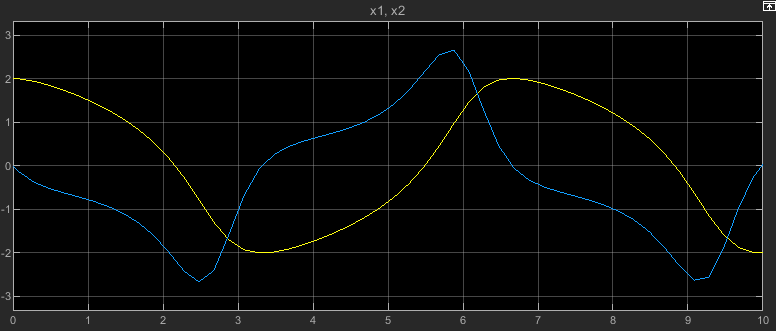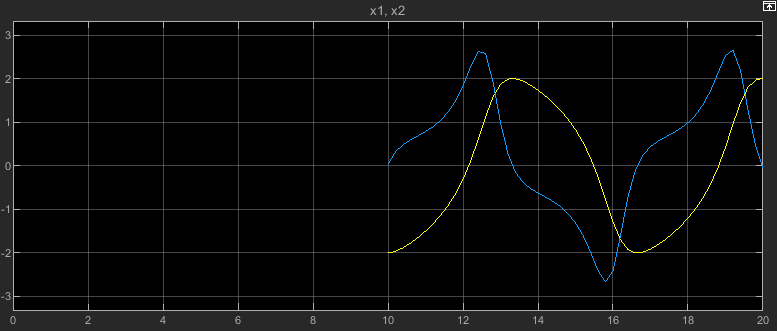Complete information that represents model operating point in simulation

Since R2019a

## Description

A `Simulink.op.ModelOperatingPoint` object contains a complete representation of a model operating point that you can use to specify the initial state for a simulation. For example, when you run a set of simulations for a model of a system that has a startup phase or initialization behavior, you can simulate the model through the end of the startup or initialization phase once, save the model operating point, then run a set of simulations that use the operating point as the initial state. If you do not modify the model, simulating a model using a `Simulink.op.ModelOperatingPoint` object as the initial state produces the same results as a simulation with no initial state specified.

The operating point for a model represents the model state in simulation, including logged block states, hidden block states, the state of the solver and execution engine, and output values for some blocks. For more information, see Use Model Operating Point for Faster Simulation Workflow.

## Creation

A model configured to save the final operating point creates a `Simulink.op.ModelOperatingPoint` object when the simulation completes or when you pause or stop the simulation. To configure a model to save the final operating point:

1. On the Modeling tab, click Model Settings.

2. In the Configuration Parameters dialog box, select Data Import/Export.

By default, the `Simulink.op.ModelOperatingPoint` object is saved using a variable called `xFinal`. To save the operating point using a different variable name, specify the variable name you want to use in the Final states text box.

When you return simulation results using a single simulation output, the `Simulink.op.ModelOperatingPoint` object is returned as part of the `Simulink.SimulationOutput` object that contains all the simulation data.

## Properties

expand all

Description of saved operating point, specified as a string or a character vector. The `ModelOperatingPoint` object has a default description that includes the name of the model and the simulation time at which the operating point was saved.

Example: ```Operating point of the model 'vdp' at simulation time 3.2137655751098118```

#### Tips

The simulation time at which the model operating point was saved is also stored in the `snapshotTime` property.

Operating point information for built-in blocks, specified as a `Simulink.SimulationData.Dataset` object or a structure.

The format of the data in the `loggedStates` property for a `ModelOperatingPoint` object created from simulation depends on the format used for logging states and output data. To configure the format for logging states and outputs, use the Configuration Parameters dialog box. On the Modeling tab, click Model Settings. Then, on the Data Import/Export pane, select a value for the Format parameter.

Format parameter valueFormat of `loggedStates` property value

`Dataset`

• `Structure with time`

• `Structure`

• `Array`

Structure that contains block states data

#### Tips

• The `loggedStates` property contains the operating point information for built-in blocks and S-functions that do not implement custom operating point behavior. To access or modify the operating point information for a Stateflow® chart, a MATLAB System block, or an S-function with a custom operating point implementation, use the `get` and `set` functions.

• Not all operating point information for built-in blocks is exposed in the `loggedStates` property for viewing or editing. For example, the `loggedStates` property does not include operating point information for:

• Blocks inside For Each subsystems

• Simscape™ blocks

• Blocks inside referenced models that execute in accelerator mode

• You cannot change the format for the data in the `loggedStates` property after a `ModelOperatingPoint` object is created.

Simulation time at which operating point was saved, returned as a double.

Start time of simulation that created operating point, returned as a double.

## Object Functions

 `get` Get operating point information for Stateflow chart, MATLAB System block, or S-function `set` Set operating point information for Stateflow chart, MATLAB System block, or S-function

## Examples

collapse all

Open the model `vdp`.

```mdl = "vdp"; open_system(mdl);```

Configure the model to save the final operating point at the end of simulation.

1. On the Modeling tab, under Setup, click Model Settings.

2. In the Configuration Parameters dialog box, select the Data Import/Export pane.

3. On the Data Import/Export tab, select Final states and Save final operating point.

4. Click OK.

Alternatively, create a `Simulink.SimulationInput` object to store the parameter values for the simulation. Then, use the `setModelParameter` function to specify the parameter values to use in the simulation.

```simIn = Simulink.SimulationInput(mdl); simIn = setModelParameter(simIn,"SaveFinalState","on"); simIn = setModelParameter(simIn,"SaveOperatingPoint","on");```

Set the stop time for the simulation to 10 seconds. On the Simulation tab, under Simulate, in the Stop Time box, enter `10`, or use the `setModelParameter` function to specify the value of the `StopTime` parameter for the simulation.

`simIn = setModelParameter(simIn,"StopTime","10");`

Simulate the model.

`out = sim(simIn);`

To view the simulation results, double-click the Scope block in the model. The plot in the Scope displays the values of the signals `x1` and `x2` over the 10-second simulation.Resume the simulation by using the operating point you saved at the end of the first simulation as the initial operating point for the second simulation.

Get the final operating point from the first simulation from the `Simulink.SimulationOutput` object `out`.

`vdpOP = out.xFinal;`

Specify the operating point as the initial state for the simulation.

1. On the Modeling tab, under Setup, click Model Settings.

2. In the Configuration Parameters dialog box, on the Data Import/Export pane, select Initial state.

3. In the text box, enter `vdpOP`.

4. Click OK.

Alternatively, create another `Simulink.SimulationInput` object to configure this simulation. Then, use the `setInitialState` function to specify the initial state.

```simIn2 = Simulink.SimulationInput(mdl); simIn2 = setInitialState(simIn2,vdpOP);```

Set the stop time for this simulation to 20. On the Simulation tab, under Simulate, in the Stop Time box, enter `20`, or use the `setModelParameter` function to specify the value of the `StopTime` parameter for the simulation.

`simIn2 = setModelParameter(simIn2,"StopTime","20");`

Simulate the model again, resuming the prior simulation by starting this simulation from the final operating point saved in the first simulation.

`out2 = sim(simIn2);`

The plot in the Scope window updates to show the data from this simulation. The time axis starts at `0` and goes to the simulation stop time of `20`. Because this simulation started from the initial operating point from the first simulation, which ended after 10 simulation seconds, the plot shows the values of the signals `x1` and `x2` only between simulation time 10 seconds and 20 seconds.## Tips

• When you load an operating point as the initial state for a simulation, the software disables the Block reduction parameter for the model. For best results, clear the Block reduction parameter before running a simulation in which you save a model operating point. If the Block reduction parameter was enabled when the operating point object was saved, you might not be able to load the operating point.

• When you use a model operating point as the initial state for a simulation that uses a fixed-step solver with the Fixed-step size (fundamental sample time) parameter set to `auto`, the software uses the fixed step size saved in the model operating point object.

• When you use a model operating point as the initial state for a simulation that uses a variable-step solver with the Max step size parameter set to `auto`, the software uses the maximum step size saved in the model operating point object.

## Version History

Introduced in R2019a

expand all